# Barplot using seaborn in Python

• Difficulty Level : Easy
• Last Updated : 10 Jun, 2021

Seaborn is an amazing visualization library for statistical graphics plotting in Python. It provides beautiful default styles and color palettes to make statistical plots more attractive. It is built on the top of matplotlib library and also closely integrated to the data structures from pandas.

### Seaborn.barplot()

seaborn.barplot() method is used to draw a barplot. A bar plot represents an estimate of central tendency for a numeric variable with the height of each rectangle and provides some indication of the uncertainty around that estimate using error bars.

Syntax : seaborn.barplot(x=None, y=None, hue=None, data=None, order=None, hue_order=None, estimator=<function mean at 0x7fa4c4f67940>, ci=95, n_boot=1000, units=None, seed=None, orient=None, color=None, palette=None, saturation=0.75, errcolor=’.26′, errwidth=None, capsize=None, dodge=True, ax=None, **kwargs)
Parameters : This method is accepting the following parameters that are described below :

• x, y : This parameter take names of variables in data or vector data, Inputs for plotting long-form data.
• hue : (optional) This parameter take column name for colour encoding.
• data : (optional) This parameter take DataFrame, array, or list of arrays, Dataset for plotting. If x and y are absent, this is interpreted as wide-form. Otherwise it is expected to be long-form.
• color : (optional) This parameter take matplotlib color, Color for all of the elements, or seed for a gradient palette.

Returns : Returns the Axes object with the plot drawn onto it.

Grouping variables in Seaborn barplot with different attributes

Example 1: Draw a set of vertical bar plots grouped by a categorical variable.
Creating a simple bar plot using seaborn.

Syntax:

seaborn.barplot( x, y, data)

## Python3

 `# importing the required library``import` `seaborn as sns``import` `matplotlib.pyplot as plt` `# read a titanic.csv file``# from seaborn library``df ``=` `sns.load_dataset(``'titanic'``)` `# class v / s fare barplot``sns.barplot(x ``=` `'class'``, y ``=` `'fare'``, data ``=` `df)` `# Show the plot``plt.show()`

Output :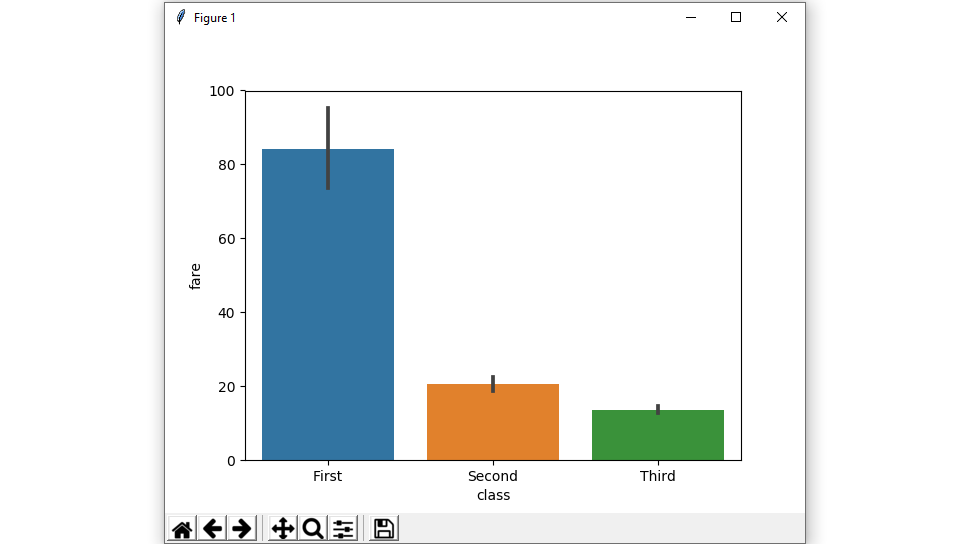Example 2: Draw a set of vertical bars with nested grouping by two variables.

Creating a bar plot using hue parameter with two variables.

Syntax:

seaborn.barplot( x, y, data, hue)

## Python3

 `# importing the required library``import` `seaborn as sns``import` `matplotlib.pyplot as plt` `# read a titanic.csv file``# from seaborn library``df ``=` `sns.load_dataset(``'titanic'``)` `# class v / s fare barplot``sns.barplot(x ``=` `'class'``, y ``=` `'fare'``, hue ``=` `'sex'``, data ``=` `df)` `# Show the plot``plt.show()`

Output :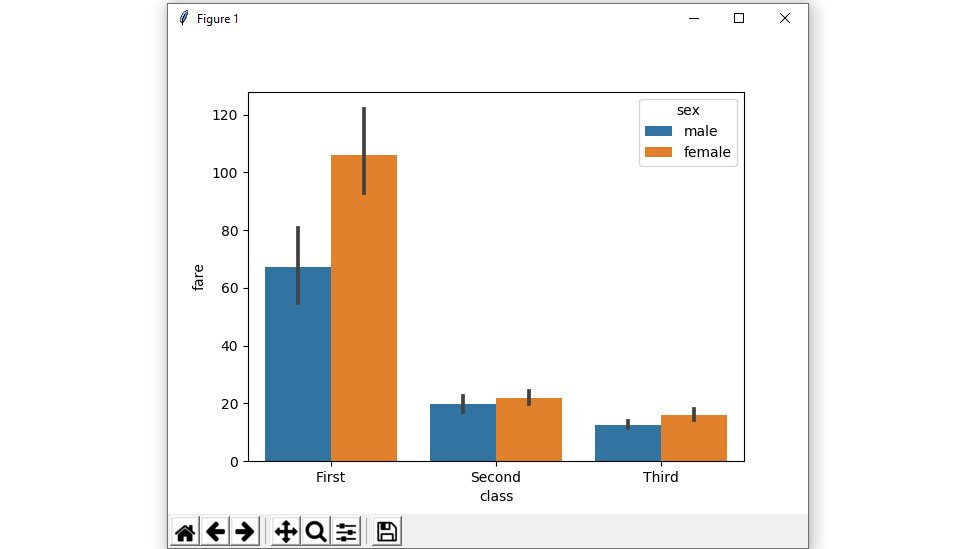Example 3: shows a Horizontal barplot.

exchange the data variable instead of two data variables then it means that the axis denotes each of these data variables as an axis.

X denotes an x-axis and y denote a y-axis.

## Python3

 `# importing the required library``import` `seaborn as sns``import` `matplotlib.pyplot as plt` `# read a titanic.csv file``# from seaborn library``df ``=` `sns.load_dataset(``'titanic'``)` `# fare v / s class horizontal barplot``sns.barplot(x ``=` `'fare'``, y ``=` `'class'``, hue ``=` `'sex'``, data ``=` `df)` `# Show the plot``plt.show()`

Output :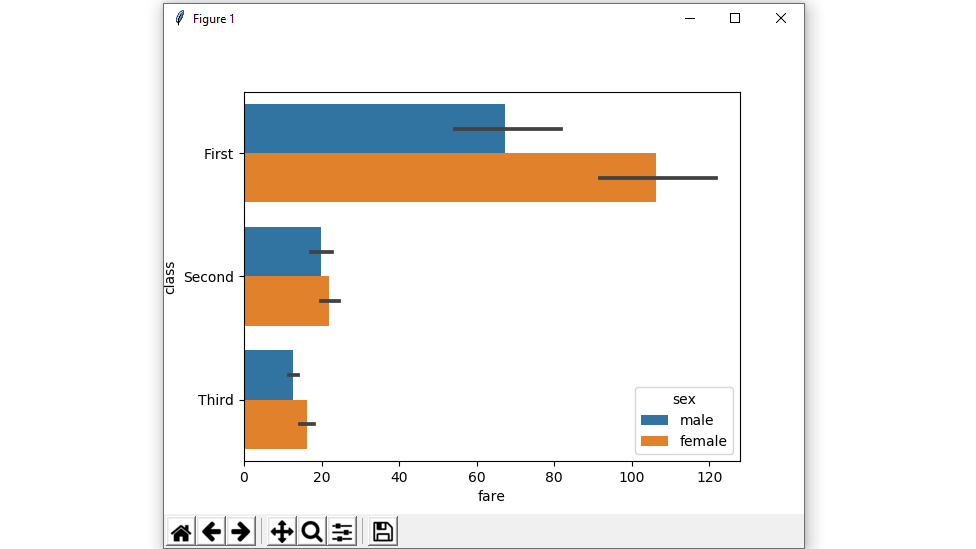Example 4: Plot all bars in a given order.

Control barplot order by passing an explicit order.

## Python3

 `# importing the required library``import` `seaborn as sns``import` `matplotlib.pyplot as plt` `# read a titanic.csv file``# from seaborn library``df ``=` `sns.load_dataset(``'titanic'``)` `# class v / s fare barplot in given order``sns.barplot(x ``=` `'class'``, y ``=` `'fare'``, data ``=` `df,``            ``order ``=` `[``"Third"``, ``"Second"``, ``"First"``])` `# Show the plot``plt.show()`

Output :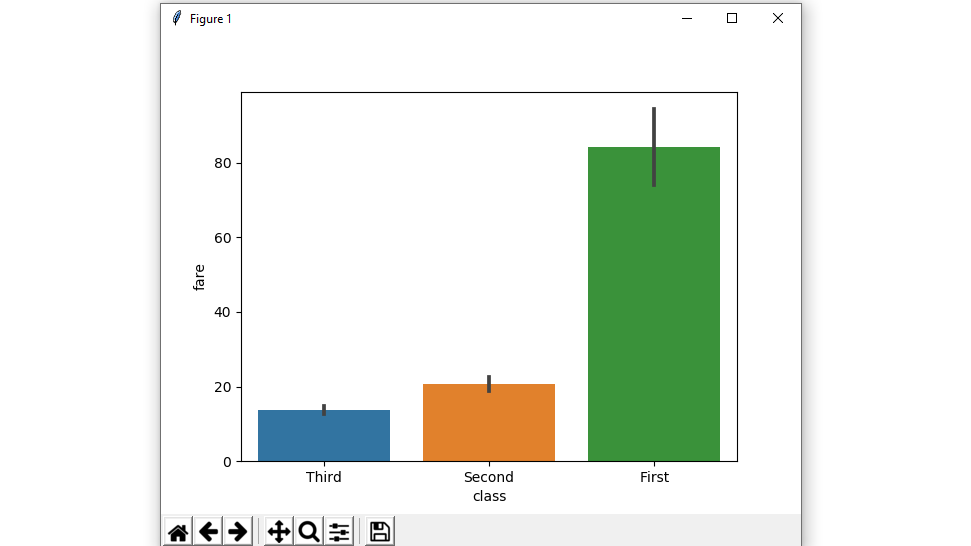Example 5: Plot all bars in a single color using color attributes.

Color for all of the elements.

Syntax:

seaborn.barplot( x, y, data, color)

## Python3

 `# importing the required library``import` `seaborn as sns``import` `matplotlib.pyplot as plt` `# read a titanic.csv file from seaborn library``df ``=` `sns.load_dataset(``'titanic'``)` `# class v / s fare barplot with same colour``sns.barplot(x ``=` `'class'``, y ``=` `'fare'``, data ``=` `df, color ``=` `"salmon"``)` `# Show the plot``plt.show()`

Output :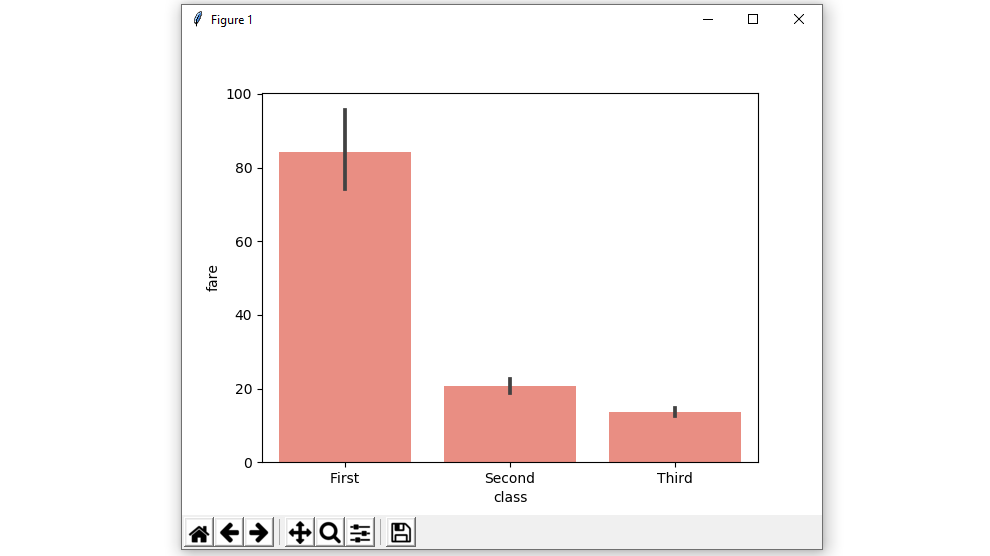Example 6: barplot without error bars using ci attributes.

We will use None it means no bootstrapping will be performed, and error bars will not be drawn

Syntax:

seaborn.barplot( x, y, data, ci)

## Python3

 `# importing the required library``import` `seaborn as sns``import` `matplotlib.pyplot as plt` `# read a titanic.csv file``# from seaborn library``df ``=` `sns.load_dataset(``'titanic'``)` `# class v / s fare barplot``# without error bars``sns.barplot(x ``=` `'class'``, y ``=` `'fare'``, data ``=` `df, ci ``=` `None``)` `# Show the plot``plt.show()`

Output :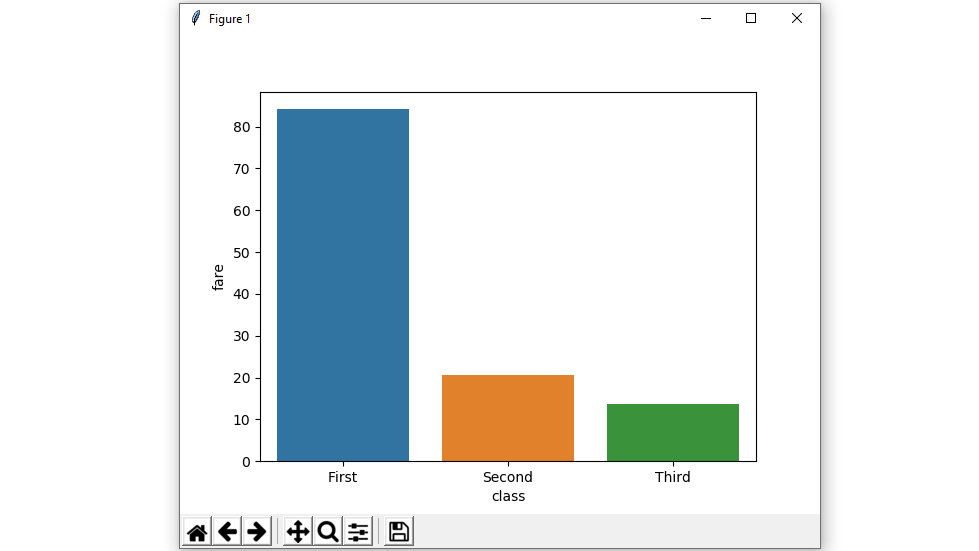Example 7: Colors to use for the different levels of the hue variable using palette.

Using the palette we can generate the point with different colors. In this below example we can see the palette can be responsible for a generate the barplot with different colormap values.

Syntax:

seaborn.barplot( x, y, data, palette=”color_name”)

## Python3

 `# importing the required library``import` `seaborn as sns``import` `matplotlib.pyplot as plt` `# read a titanic.csv file``# from seaborn library``df ``=` `sns.load_dataset(``'titanic'``)` `sns.barplot(x ``=` `'class'``, y ``=` `'fare'``,``            ``hue ``=` `'sex'``, data ``=` `df, palette``=``'pastel'``)` `# Show the plot``plt.show()`

Output: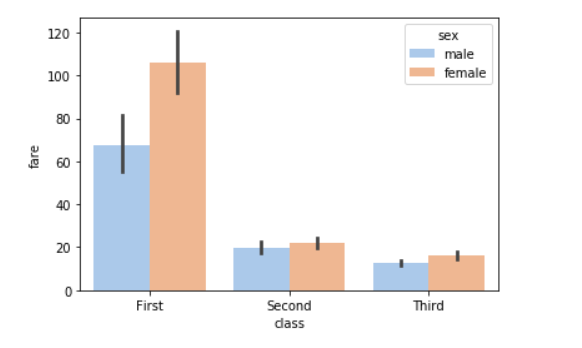Possible values of palette are:

Accent, Accent_r, Blues, Blues_r, BrBG, BrBG_r, BuGn, BuGn_r, BuPu, BuPu_r, CMRmap, CMRmap_r, Dark2, Dark2_r,

GnBu, GnBu_r, Greens, Greens_r, Greys, Greys_r, OrRd, OrRd_r, Oranges, Oranges_r, PRGn, PRGn_r, Paired, Paired_r,

Pastel1, Pastel1_r, Pastel2, Pastel2_r, PiYG, PiYG_r, PuBu, PuBuGn, PuBuGn_r, PuBu_r, PuOr, PuOr_r, PuRd, PuRd_r,

Purples, Purples_r, RdBu, RdBu_r, RdGy, RdGy_r, RdPu, RdPu_r, RdYlBu, RdYlBu_r, RdYlGn, RdYlGn_r, Reds, Reds_r, Set1,

Set1_r, Set2, Set2_r, Set3, Set3_r, Spectral, Spectral_r, Wistia, Wistia_r, YlGn, YlGnBu, YlGnBu_r, YlGn_r, YlOrBr,

YlOrBr_r, YlOrRd, YlOrRd_r, afmhot, afmhot_r, autumn, autumn_r, binary, binary_r, bone, bone_r, brg, brg_r, bwr, bwr_r,

cividis, cividis_r, cool, cool_r, coolwarm, coolwarm_r, copper, copper_r, cubehelix, cubehelix_r, flag, flag_r, gist_earth,

gist_earth_r, gist_gray, gist_gray_r, gist_heat, gist_heat_r, gist_ncar, gist_ncar_r, gist_rainbow, gist_rainbow_r, gist_stern,

Example 8: Using the statistical function NumPy.median and NumPy.mean to estimate within each categorical bin.

## Python3

 `# importing the required library``import` `seaborn as sns``from` `numpy ``import` `median``import` `matplotlib.pyplot as plt` `# read a titanic.csv file``# from seaborn library``df ``=` `sns.load_dataset(``'titanic'``)` `sns.barplot(x ``=` `'class'``, y ``=` `'fare'``, hue ``=` `'sex'``, data ``=` `df, estimator``=``median)` `# Show the plot``plt.show()`

Output: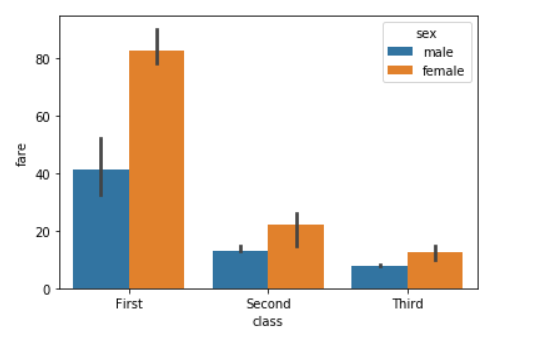For Numpy.mean:

## Python3

 `from` `numpy ``import` `mean``sns.barplot(x ``=` `'class'``, y ``=` `'fare'``, hue ``=` `'sex'``, data ``=` `df, estimator``=``mean)`

Output: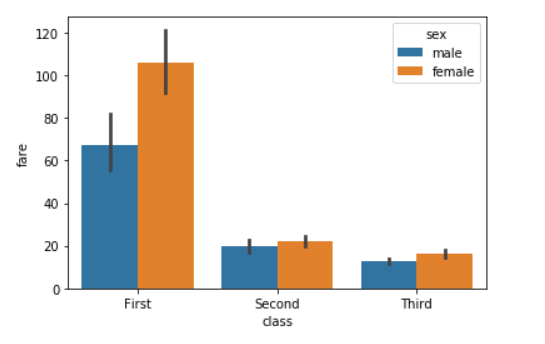Example 9: Using the saturation parameter.

The proportion of the original saturation to draw colors at. Large patches often look better with slightly desaturated colors, but set this to 1 if you want the plot colors to perfectly match the input color spec.

Syntax:

seaborn.barplot( x, y, data, saturation)

## Python3

 `# importing the required library``import` `seaborn as sns``import` `matplotlib.pyplot as plt` `# read a titanic.csv file``# from seaborn library``df ``=` `sns.load_dataset(``'titanic'``)` `sns.barplot(x ``=` `'class'``, y ``=` `'fare'``, hue ``=` `'sex'``, data ``=` `df,saturation ``=` `0.1``)` `# Show the plot``plt.show()`

Output: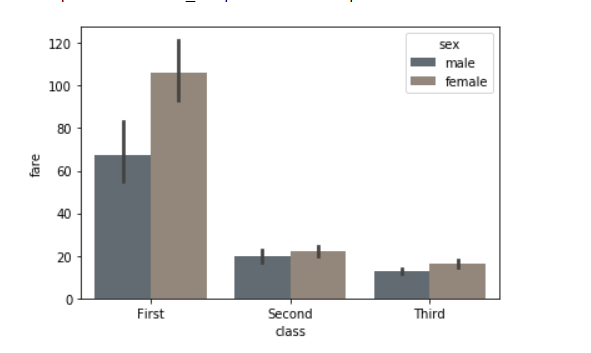Example 10: Use matplotlib.axes.Axes.bar() parameters to control the style.

We can set Width of the gray lines that frame the plot elements using linewidth. Whenever we increase linewidth than the point also will increase automatically.

Syntax:

seaborn.barplot(x, y, data, linewidth, edgecolor)

## Python3

 `# importing the required library``import` `seaborn as sns``import` `matplotlib.pyplot as plt` `# read a titanic.csv file``# from seaborn library``df ``=` `sns.load_dataset(``'titanic'``)` `sns.barplot(x``=``"class"``, y``=``"fare"``, data``=``df,``                 ``linewidth``=``2.5``, facecolor``=``(``1``, ``1``, ``1``, ``0``),``                 ``errcolor``=``".2"``, edgecolor``=``".2"``)`

Output: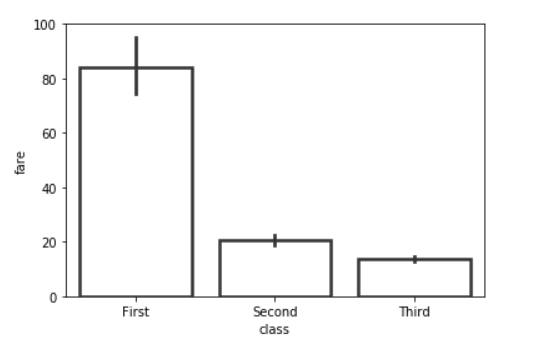My Personal Notes arrow_drop_up